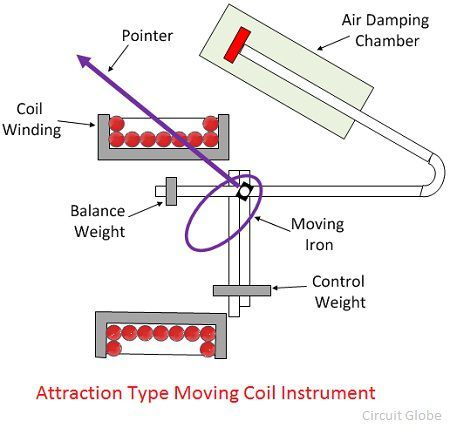# Moving Iron or MI Instrument

Definition: The instrument in which the moving iron is used for measuring the flow of current or voltage is known as the moving iron instrument. It works on the principle that the iron place near the magnet attracts towards it. The force of attraction depends on the strength of the magnet field. The magnetic field induces by the electromagnet whose strength depends on the magnitude of the current passes through it.

## Construction of Moving Iron Instrument

The plate or vane of soft iron is used as the moving element of the instrument. The vane is so placed that it can freely move in the magnetic field of the stationary coil. The conductor makes the stationary coil, and it is excited by the voltage or current whose magnitude is used to be measured.

The moving iron instrument uses the stationary coil as an electromagnet. The electromagnet is the temporary magnet whose magnetic field strength increases or decreases with the magnitude of the current passes through it.

## Working of the Moving Iron Instrument

The moving iron instruments use the stationary coil of copper or aluminium wire which acts as an electromagnet when an electric current passes through it. The strength of the magnetic field induces by the electromagnet is directly proportional to the current passes through it.

The plates or vane of the iron pass through the coil increases the inductance of the stationary coil (the inductance is the property of the conductor which increases their electromotive force when the varying current passes through it).

The electromagnet attracts the iron vane. The vane passes through the coil tries to occupy the minimum reluctance path (the reluctance is the property of the magnet which opposes the flow of electric current).

The vane passes through the coil experience a force of repulsion caused by the electromagnet. The repulsion force increases the strength of the coil inductance.
This happens because the inductance and reluctances are inversely proportional to each other.

### Classification of the Moving Iron Instruments

The attraction and the repulsion are the types of the moving iron instruments. Their detail explanation is shown below.

1. Attraction Type – The instrument in which the iron plate attracts from the weaker field towards the stronger field such type of instrument is known as the attraction type instrument.

Construction of Attraction Type Instrument – The stationary coil of the attraction type instrument is flat and has a narrow opening. The moving element is the flat disc of the iron core. The current flow through the stationary coil produced the magnetic field which attracts the iron coil.The iron vane deflects from the low magnetic field to the high magnetic field, and the strength of the deflection is directly proportional to the magnitude of the current flow through it. In short, we can say that the iron coil attracts towards in.

The attraction type instruments use spring, which provided the controlling torque. The deflection of the coil is reduced by the aluminium piston which is attached to the moving coil.

2. Repulsion Type Instruments – The repulsion type instrument has two vanes or iron plates. One is fixed, and the other one is movable. The vanes become magnetised when the current passes through the stationary coil and the force of repulsion occur between them. Because of a repulsive force, the moving coil starts moving away from the fixed vane.The spring provides the controlling torque. The air friction induces the damping torque, which opposes the movement of the coil. The repulsion type instrument is a non-polarized instrument, i.e., free from the direction of current passes through it. Thus, it is used for both AC and DC.

### Advantages of the MI Instruments

The following are the advantages of the moving iron instruments.

1. Universal use – The MI instrument is independent of the direction of current and hence used for both AC and DC.
2. Less Friction Error  The friction error is very less in the moving iron instrument because their torque weight ratio is high. The torque weight ratio is high because their current carrying part is stationary and the moving parts are lighter in weight.
3. Cheapness – The MI instruments require less number of turns as compared to PMMC instrument. Thus, it is cheaper.
4. Robustness – The instrument is robust because of their simple construction. And also because their current carrying part is stationary.

### Disadvantages of Moving Iron Instruments.

The following are the disadvantages of Moving Iron Instrument.

1. Accuracy – The scale of the moving iron instruments is not uniform, and hence the accurate result is not possible.
2. Errors – Some serious error occurs in the instruments because of the hysteresis, frequency and stray magnetic field.
3. Waveform Error –  In MI instrument the deflection torque is not directly proportional to the square of the current. Because of which the waveforms error occurs in the instrument.
4. Difference between AC and DC calibration –  The calibration of the AC and DC are differed because of the effect of the inductance of meter and the eddy current which is used on AC. The AC is calibrated on the frequency at which they use.

Two type of error occurs in the MI instruments i.e., the error which occurs on both AC and DC and the error which only occur on AC.